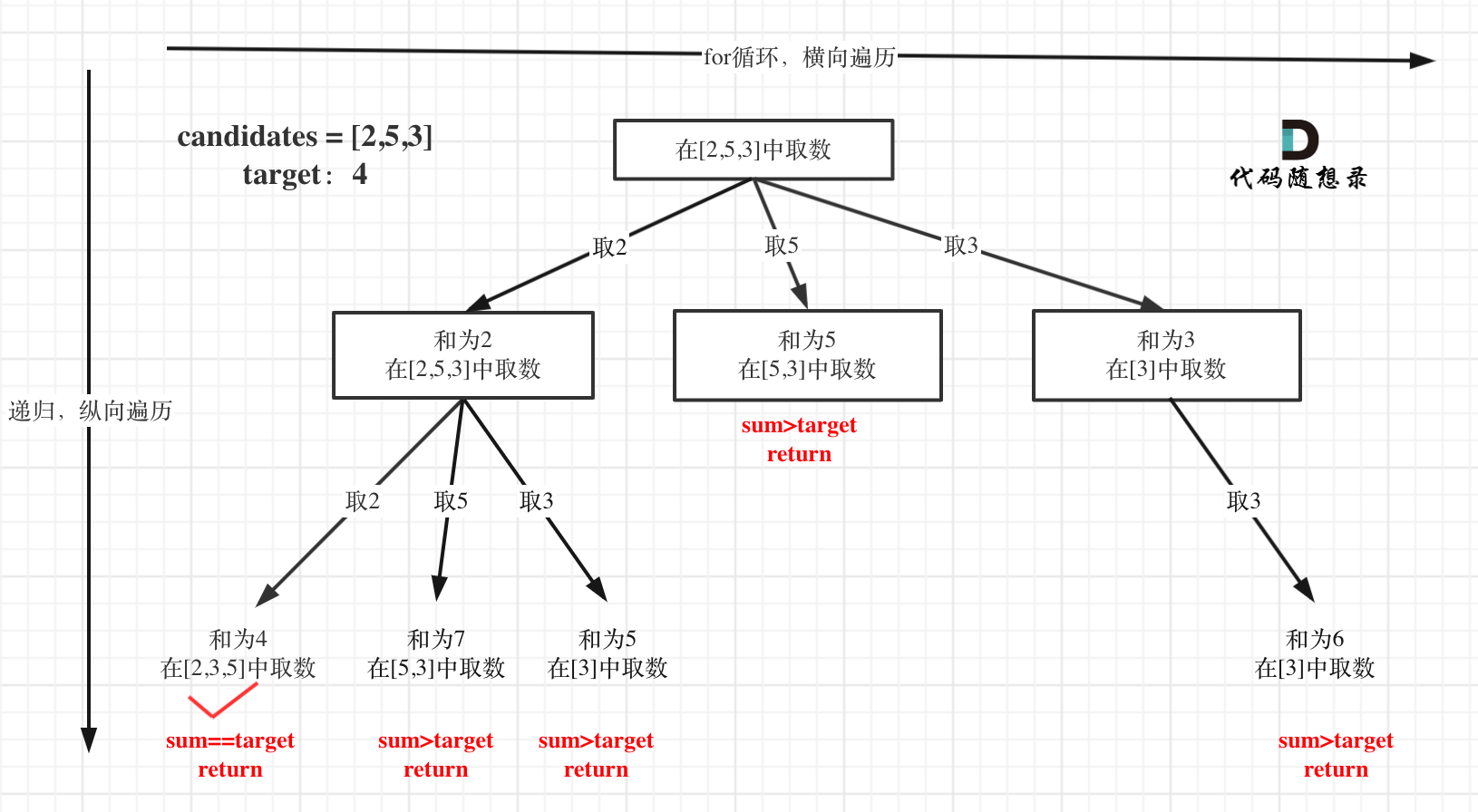# Leetcode 39 - Combination sum

Note:

• This is a backtracking problem.
• The key is to make every element get added multi times.
• We still need startIndex because we need it to know where we should start our forloop. If we just use 0 for start point, there will be duplicates. Like [2,5,3] vs [5,2,3].

Given an array of distinct integers candidates and a target integer target, return a list of all unique combinations of candidates where the chosen numbers sum to target. You may return the combinations in any order.

The same number may be chosen from candidates an unlimited number of times. Two combinations are unique if the frequency of at least one of the chosen numbers is different.

It is guaranteed that the number of unique combinations that sum up to target is less than 150 combinations for the given input.

Example